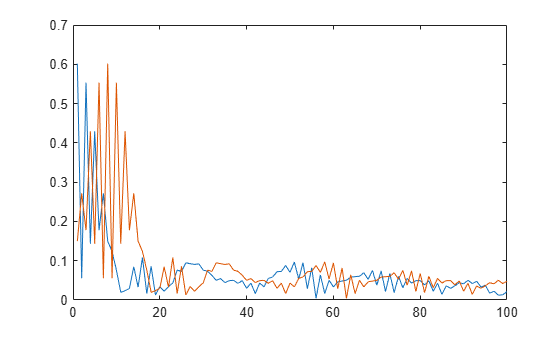# lteULFrameOffsetPUCCH1

PUCCH format 1 DM-RS uplink subframe timing estimate

## Syntax

``offset = lteULFrameOffsetPUCCH1(ue,chs,waveform)``
``````[offset,corr] = lteULFrameOffsetPUCCH1(ue,chs,waveform)``````

## Description

example

````offset = lteULFrameOffsetPUCCH1(ue,chs,waveform)` performs synchronization using PUCCH format 1 demodulation reference signals (DM-RS) for the time-domain waveform, `waveform`, given UE-specific settings, `ue`, and PUCCH format 1 configuration, `chs`.The returned value `offset` indicates the number of samples from the start of the waveform `waveform` to the position in that waveform where the first subframe containing the DM-RS begins.`offset` provides subframe timing. Frame timing can be achieved by using `offset` with the subframe number, `ue``.NSubframe`. This behavior is consistent with real-world operation because the base station knows when, or in which subframe, to expect uplink transmissions.```

example

``````[offset,corr] = lteULFrameOffsetPUCCH1(ue,chs,waveform)``` also returns a complex matrix `corr`, which is the signal used to extract the timing offset. ```

## Examples

collapse all

Synchronize and demodulate a transmission that has been delayed by four samples using the PUCCH format 1 demodulation reference signal (DM-RS) symbols.

Initialize configuration structures (`ue` and `pucch1`).

```ue = struct('NULRB',6,'NCellID',0,'NSubframe',0,'Hopping','Off'); ue.CyclicPrefixUL = 'Normal'; ue.NTxAnts = 1; pucch1 = struct('ResourceIdx',0); pucch1.CyclicShifts = 0; pucch1.DeltaShift = 1; pucch1.ResourceSize = 0;```

On the transmit side, populate `reGrid`, generate `waveform`, and insert a delay of four samples.

```reGrid = lteULResourceGrid(ue); reGrid(ltePUCCH1DRSIndices(ue,pucch1)) = ltePUCCH1DRS(ue,pucch1); waveform = lteSCFDMAModulate(ue,reGrid); tx = [zeros(4,1); waveform];```

On the receive side, perform synchronization using the PUCCH format 1 DM-RS symbols for the time-domain waveform and demodulate adjusting for the frame timing estimate. Show estimated frame timing offset.

`fOffset = lteULFrameOffsetPUCCH1(ue,pucch1,tx)`
```fOffset = 4 ```
`rxGrid = lteSCFDMADemodulate(ue,tx(1+fOffset:end));`

View the correlation peak for a delayed transmit waveform. The transmission contains PUCCH format 1 demodulation reference signal (DM-RS) symbols available for estimating the waveform timing.

UE Configuration

Configure UE-specific settings and channel transmission parameters.

```ue = struct('NULRB',6,'NCellID',0,'NSubframe',0,'Hopping','Off'); ue.CyclicPrefixUL = 'Normal'; ue.NTxAnts = 1; pucch1 = struct('ResourceIdx',0,'CyclicShifts',0, ... 'DeltaShift',1,'ResourceSize',0);```

Generate Transmit Waveform

On the transmit side, populate a resource grid and generate a waveform containing PUCCH1 DM-RS.

```reGrid = lteULResourceGrid(ue); reGrid(ltePUCCH1DRSIndices(ue,pucch1)) = ltePUCCH1DRS(ue,pucch1); tx = lteSCFDMAModulate(ue,reGrid);```

Waveform Reception

On the receive side, calculate timing offset using the PUCCH format 1 DM-RS symbols for the time-domain waveform. Estimate the correlations for the transmit waveform and for a delayed version of the transmit waveform.

```[~,corr] = lteULFrameOffsetPUCCH1(ue,pucch1,tx); txDelayed = [zeros(7,1); tx]; [offset,corrDelayed] = lteULFrameOffsetPUCCH1(ue,pucch1,txDelayed);```

Plot the correlation data before and after delay is added. Zoom in on the x-axis to view correlation peaks.

```plot(corr) hold on plot(corrDelayed) hold off xlim([0 100])```Correct the timing offset and demodulate the received waveform.

`rxGrid = lteSCFDMADemodulate(ue,txDelayed(1+offset:end));`

## Input Arguments

collapse all

UE-specific settings, specified as a scalar structure with the following fields.

Parameter FieldRequired or OptionalValuesDescription
`NULRB`Required

Scalar integer from 6 to 110

Number of uplink resource blocks. (${N}_{\text{RB}}^{\text{UL}}$)

`NCellID`Required

Integer from 0 to 503

Physical layer cell identity

`NSubframe`Required

0 (default), nonnegative scalar integer

Subframe number

`CyclicPrefixUL`Optional

`'Normal'` (default), `'Extended'`

Cyclic prefix length

`NTxAnts`Optional

1 (default), 2, 4

Number of transmission antennas.

`Hopping`Optional

`'Off'` (default), `'Group'`

Frequency hopping method.

`NPUCCHID`Optional

Integer from 0 to 503

PUCCH virtual cell identity. If this field is not present, `NCellID` is used as the identity.

Data Types: `struct`

PUCCH format 1 configuration, specified as a scalar structure with the following fields.

Parameter FieldRequired or OptionalValuesDescription
`ResourceIdx`Optional

0 (default), integer from 0 to 2047 or vector of integers.

PUCCH resource indices, specified as an integer or a vector of integers. Values range from 0 to 2047. These indices determine the physical resource blocks, cyclic shift and orthogonal cover used for transmission. (${n}_{PUCCH}^{\left(1\right)}$). Define one index for each transmission antenna.

`ResourceSize`Optional

0 (default), integer from 0 to 98.

Size of resource allocated to PUCCH format 2 (${N}_{RB}^{\left(2\right)}$)

`DeltaShift`Optional

1 (default), 2, 3

Delta shift, specified as 1, 2, or 3. (Δshift)

`DeltaOffset`Optional

0 (default), 1, 2

(Δoffset). Warning: The use of this parameter field is not advised. It applies only to 3GPP releases preceding v8.5.0. This parameter will be removed in a future release.

`CyclicShifts`Optional

0 (default), integer from 0 to 7

Number of cyclic shifts used for format 1 in resource blocks (RBs) with a mixture of format 1 and format 2 PUCCH, specified as an integer from 0 to 7. (${N}_{cs}^{\left(1\right)}$)

Data Types: `struct`

Time-domain waveform, specified as a numeric matrix. `waveform` must be a NS-by-NR matrix, where NS is the number of time-domain samples and NR is the number of receive antennas. `waveform` should be at least one subframe long and contain the DM-RS signals.

Generate `waveform` by SC-FDMA modulation of a resource matrix using `lteSCFDMAModulate` function, or by using one of the channel model functions (`lteFadingChannel`, `lteHSTChannel`, or `lteMovingChannel`).

Data Types: `double`
Complex Number Support: Yes

## Output Arguments

collapse all

Number of samples from the start of the waveform to the position in that waveform where the first subframe containing the DM-RS begins, returned as a scalar integer. `offset` is computed by extracting the timing of the peak of the correlation between `waveform` and internally generated reference waveforms containing DM-RS signals. The correlation is performed separately for each antenna and the antenna with the strongest correlation is used to compute `offset`.

Note

`offset` is the position of `mod(max(abs(corr),LSF))`, where LSF is the subframe length.

Signal used to extract the timing offset, returned as a complex numeric matrix. `corr` has the same dimensions as `waveform`.

## Version History

Introduced in R2014a